# Increasing Squares Toothpick Challenge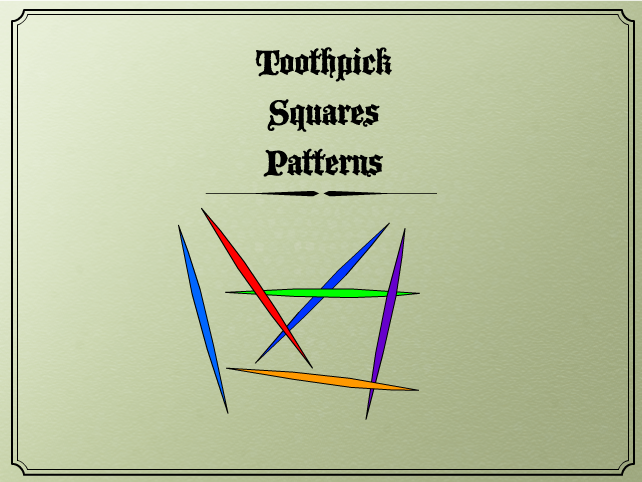What is the pattern of adding toothpicks to make squares?
-> hints.

For example: How many toothpicks to make a

• 1 x 1 square?
• 2 x 2 square?
• 3 x 3 square?
• 4 x 4 square?
• ...

How many tooth picks does it take to make one square?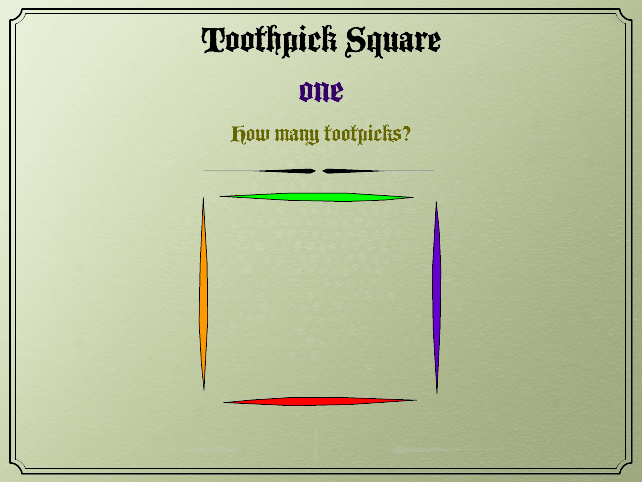How many tooth picks does it take to make a 2 x 2 square?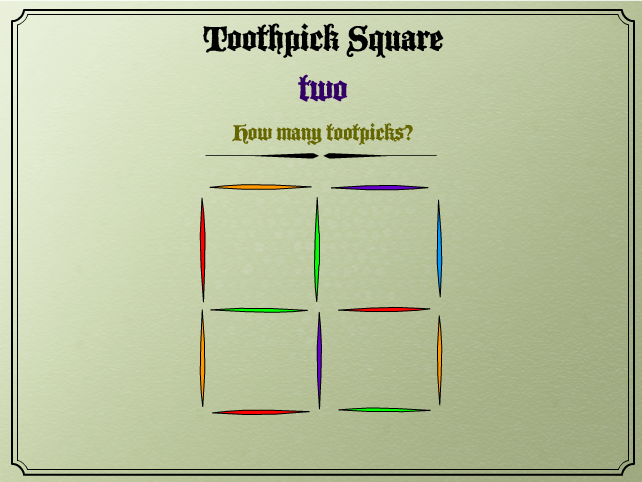How many tooth picks does it take to make a 3 x 3 square?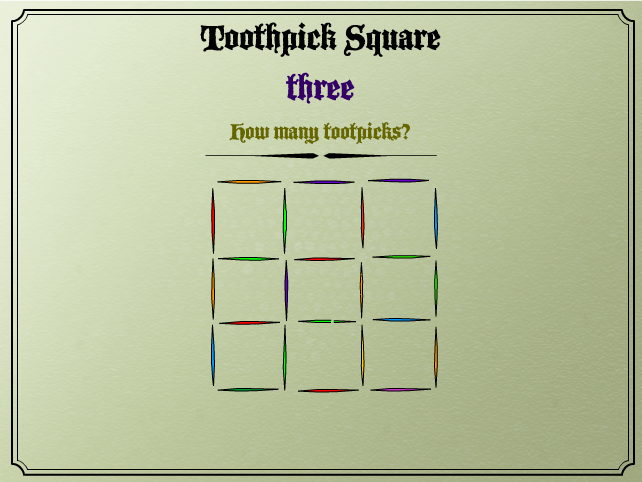Squares to infinity?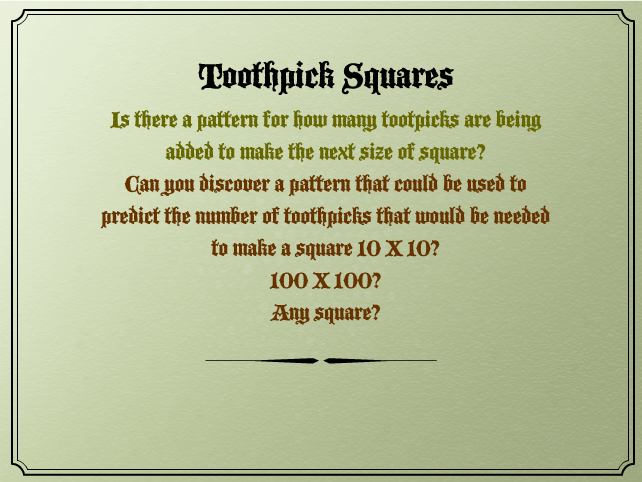Hint:

Added squares may not need four toothpicks as they use toothpicks for adjacent squares

Make a table with the results, find patterns, make a rule, function, equation ...

Discussion:

Make a table with the number of toothpicks and the number of squares. Start with 1x1 and when comfortable with understanding the progression of additional 1x1 squares move on to 2x2, and further.

Size of square

Number of squares

Number of toothpicks

1 x 1 1 4
2 x 2 4 12
3 x 3 9 24
4 x 4
5 x 5

Mind boggler: What are the different ways solutions can be considered and discovered? Try:

Enjoy!